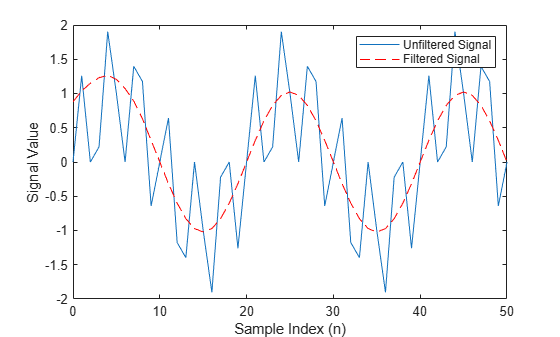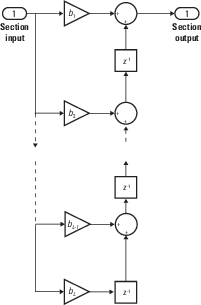# filter

1-D digital filter of `fi` objects

## Syntax

``y = filter(b,1,x)``
``[y,zf] = filter(b,1,x,zi)``
``y = filter(b,1,x,zi,dim)``

## Description

example

````y = filter(b,1,x)` filters the data in the fixed-point vector `x` using the filter described by the fixed-point vector `b`. The function returns the filtered data in the output `fi` object `y`.`filter` always operates along the first non-singleton dimension. Thus, the filter operates along the first dimension for column vectors and nontrivial matrices and along the second dimension for row vectors.```
````[y,zf] = filter(b,1,x,zi)` uses initial conditions `zi` for the filter delays. The length of `zi` must equal `length(b)-1`. The final conditions of the delays are returned in `zf`.```
````y = filter(b,1,x,zi,dim)` acts along dimension `dim`. If you do not want to specify the vector of initial conditions, use `[]` for the input argument `zi`. NoteThis function is a 1-D digital filter for `fi` objects. To filter non-`fi` data, use the MATLAB® `filter` function. ```

## Examples

collapse all

Filter a high-frequency fixed-point sinusoid from a signal that contains both a low- and high-frequency fixed-point sinusoid.

```w1 = 0.1*pi; w2 = 0.6*pi; n = 0:999; xd = sin(w1*n) + sin(w2*n); x = sfi(xd,12); b = ufi([0.1:0.1:1,1-0.1:-0.1:0.1]/4,10); gd = (length(b)-1)/2; y = filter(b,1,x);```

Plot the results, accomodating for the group delay of the filter.

```plot(n(1:end-gd),x(1:end-gd)) hold on plot(n(1:end-gd),y(gd+1:end),'r--') axis([0 50 -2 2]) legend('Unfiltered Signal','Filtered Signal') xlabel('Sample Index (n)') ylabel('Signal Value')```The resulting plot shows both the unfiltered and filtered signals.

## Input Arguments

collapse all

Filter coefficients, specified as a fixed-point vector.

Data Types: `fi`

Input data, specified as a fixed-point vector.

Data Types: `fi`

Initial conditions for filter delays, specified as a fixed-point vector. `zi` must be a `fi` object with the same data type as `y` and `zf`.

• If `zi` is a vector, then its length must be `length(b)-1`.

• If `zi` is a matrix or multidimensional array, then the size of the leading dimension must be `length(b)-1`. The size of each remaining dimension must match the size of the corresponding dimension of `x`.

If you do not specify a value for `zi`, or if you specify `[]`, it defaults to a fixed-point array with a value of `0` and the appropriate `numerictype` and size.

Data Types: `fi`

Dimension along which to operate, specified as a positive integer scalar.

Data Types: `single` | `double` | `int8` | `int16` | `int32` | `int64` | `uint8` | `uint16` | `uint32` | `uint64` | `fi`

## Output Arguments

collapse all

Filtered data, returned as a fixed-point `fi` vector, matrix, or multidimensional array.

Final conditions for filter delays, returned as a fixed-point `fi` vector, matrix, or multidimensional array.

## Tips

• The `filter` function only supports FIR filters. In the general filter representation b/a, the denominator a of an FIR filter is the scalar 1, which is the second input of this function.

• The `numerictype` of `b` can be different than the `numerictype` of `x`.

• If you want to specify initial conditions but do not know what `numerictype` to use, first try filtering your data without initial conditions. You can do so by specifying `[]` for the input `zi`. After performing the filtering operation, you have the `numerictype` of `y` and `zf` (if requested). Because the `numerictype` of `zi` must match that of `y` and `zf`, you now know the `numerictype` to use for the initial conditions.

## Algorithms

collapse all

### Filter length (L)

The filter length is `length(b)` or the number of filter coefficients specified in the fixed-point vector `b`.

### Filter order (N)

The filter order is the number of states (delays) of the filter and is equal to `L-1`.

### Direct-Form Transposed FIR Filter

The `filter` function uses a Direct-Form Transposed FIR implementation of this difference equation:

`$y\left(n\right)={b}_{1}*{x}_{n}+{b}_{2}*{x}_{n-1}+...+{b}_{L}*{x}_{n-N}$`

where L is the Filter length (L) and N is the Filter order (N).

This diagram shows the direct-form transposed FIR filter structure used by the `filter` function.### `fimath` Propagation Rules

The `filter` function uses these rules regarding `fimath` behavior:

• `globalfimath` is obeyed.

• If any of the inputs has an attached `fimath`, then it is used for intermediate calculations.

• If more than one input has an attached `fimath`, then the `fimaths` must be equal.

• The output `y` is always associated with the default `fimath`.

• If the input vector `zi` has an attached `fimath`, then the output vector `zf` retains this `fimath`.

## Version History

Introduced in R2010a